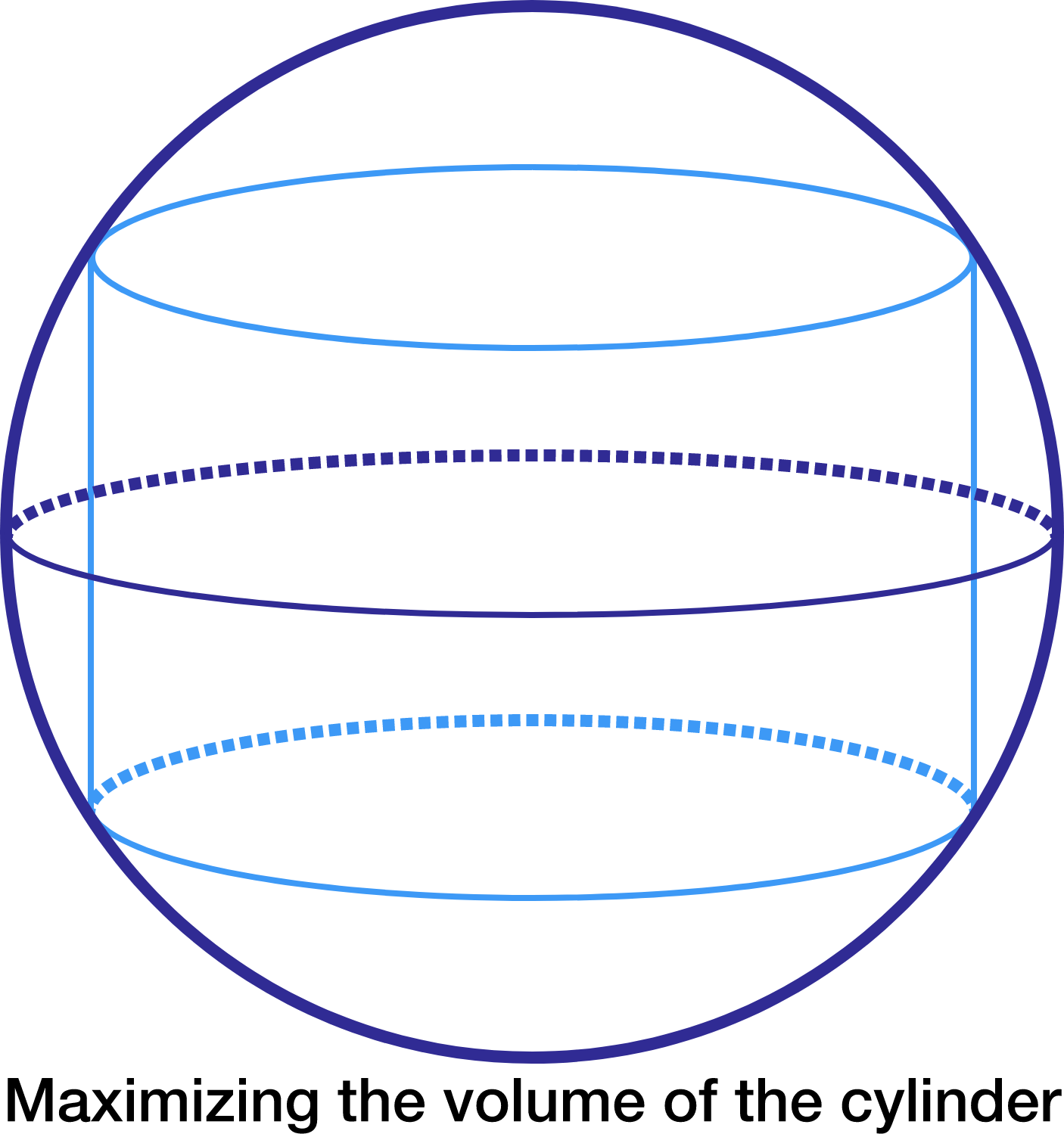# Cylinder In A Sphere

Calculus Level 4If the maximum volume of a right circular cylinder inscribed in a sphere of unit volume can be written as $\dfrac a{\sqrt b},$ where $a$ and $b$ are positive integers with $b$ square-free, find $a + b$.

×